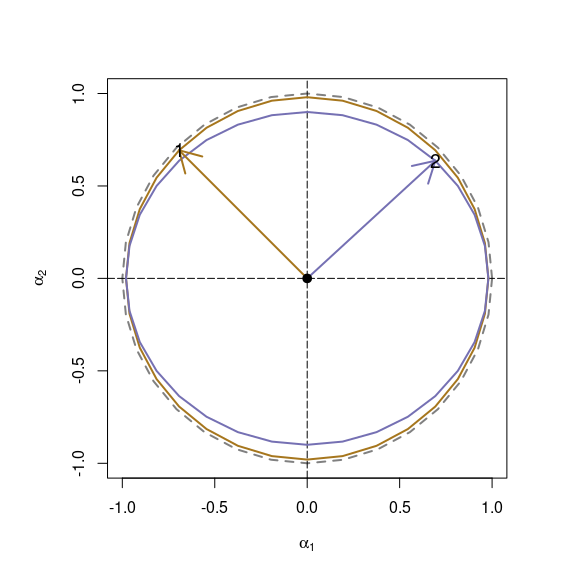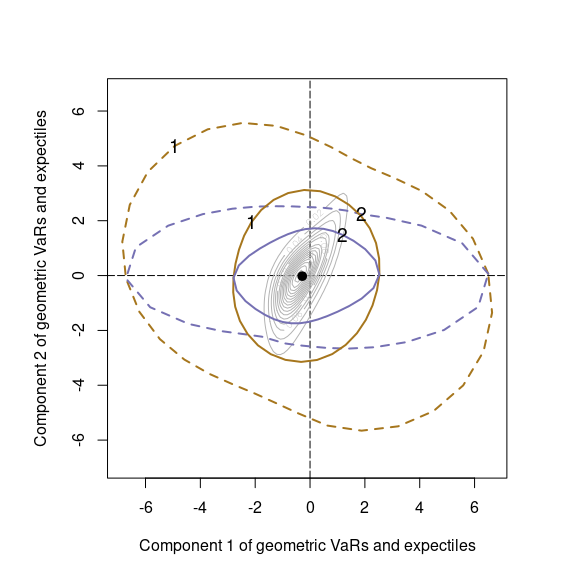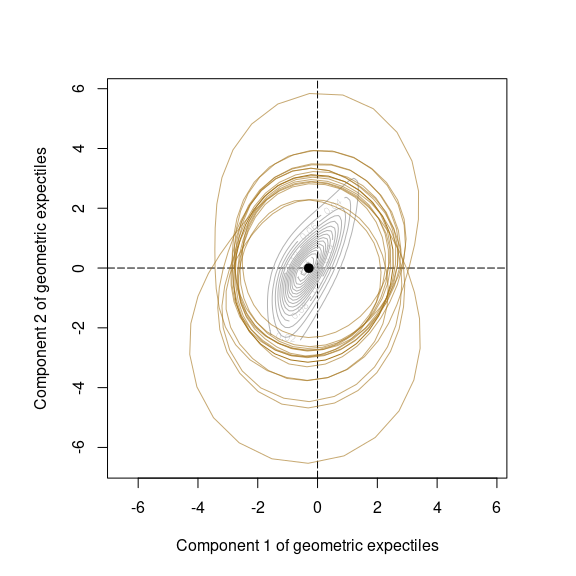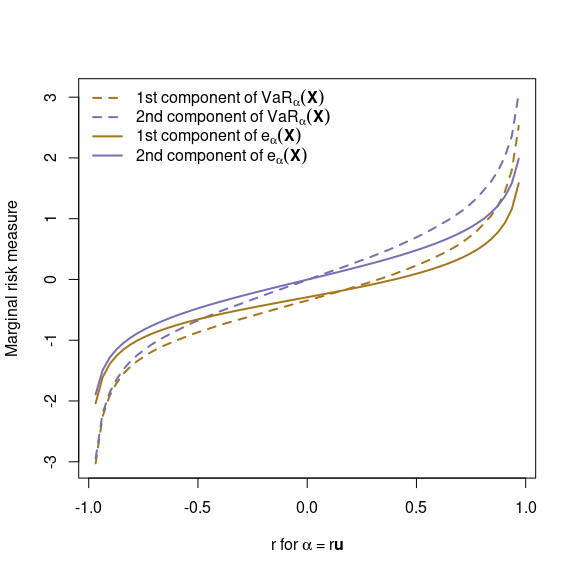# Geometric Risk Measures

#### 2020-04-19

library(qrmtools)
library(copula)
library(sn) # for skew-normal distribution
library(RColorBrewer) # for Dark2 color palette
col <- brewer.pal(8, name = "Dark2")[c(7, 3, 5, 4, 6)] # some colors

In this vignette, we briefly present some examples on geometric value-at-risk (VaR) and geometric expectiles; see Chaudhuri (1996, “On a geometric notion of quantiles for multivariate data”) and Herrmann et al. (2017, “Multivariate geometric expectiles”). Geometric VaR and geometric expectile are defined by \begin{align*} \mathrm{VaR}_{\mathbf{\alpha}}(\mathbf{X})&=\mathrm{argmin}_{\mathbf{c}\in\mathbb{R}^d}\ \mathbb{E}(\Phi_{\mathbf{\alpha}}(\mathbf{X}-\mathbf{c}))\quad\text{for}\quad\Phi_{\mathbf{\alpha}}(\mathbf{t})=(\lVert\mathbf{t}\rVert_2+\langle\mathbf{\alpha},\mathbf{t}\rangle)/2,\\ \mathrm{e}_{\mathbf{\alpha}}(\mathbf{X})&=\mathrm{argmin}_{\mathbf{c}\in\mathbb{R}^d}\ \mathbb{E}(\Lambda_{\mathbf{\alpha}}(\mathbf{X}-\mathbf{c}))\quad\text{for}\quad\Lambda_{\mathbf{\alpha}}(\mathbf{t})=\lVert\mathbf{t}\rVert_2(\lVert\mathbf{t}\rVert_2+\langle\mathbf{\alpha},\mathbf{t}\rangle)/2, \end{align*} respectively.

## 1 Geometric VaR and expectile for two sets of confidence levels

Consider the following two sets of confidence levels.

## Setup
lphi <- 33 # number of angles
phi <- seq(0, 2*pi, length.out = lphi) # equidistant angles
circle <- cbind(cos(phi), sin(phi)) # unit circle evaluated at angles
a1 <- 0.98 * circle # first set of alphas
a2 <- cbind(0.98 * circle[,1], 0.9 * circle[,2]) # other set of alphas

Here is a graphical representation. We also identify two specific points in these sets of confidence levels.

## Plot
par(pty = "s")
plot(circle, type = "l", lty = 2, lwd = 2, col = gray(0.5),
xlab = expression(alpha), ylab = expression(alpha),
xlim = c(-1, 1), ylim = c(-1, 1)) # gray circle
abline(v = 0, h = 0, lty = 5) # alpha_1 = 0 and alpha_2 = 0
lines(a1[,1], a1[,2], lwd = 2, col = col) # first set of alphas
lines(a2[,1], a2[,2], lwd = 2, col = col) # other set of alphas
p1 <- 0.98 * c(cos(3/4*pi), sin(3/4*pi)) # location of "1"
p2 <- c(0.98 * cos(1/4*pi), 0.90 * sin(1/4*pi)) # location of "2"
arrows(0, 0, p1, p1, lwd = 2, col = col) # arrow to "1"
arrows(0, 0, p2, p2, lwd = 2, col = col) # arrow to "2"
points(p1, p1, pch = "1", cex = 1.2) # "1"
points(p2, p2, pch = "2", cex = 1.2) # "2"
points(0, 0, pch = 19, cex = 1.2) # filled dotLet us generate an example data set based on which we compute geometric VaRs and geometric expectiles (see below) for the two sets of confidence levels. To keep run time small, note that we use rather small sample sizes here (and below).

## Generate a copula sample
set.seed(42) # for reproducibility
n <- 2e3 # sample size
cop <- gumbelCopula(iTau(gumbelCopula(), tau = 0.5)) # Gumbel copula
U <- rCopula(n, copula = cop) # sample
## Map to skew-normal and t_4 margins
xi <- -1; om <- 1; al <- 2 # parameters for skew-normal margin
nu <- 4 # parameter for t margin
X <- cbind(qsn(U[,1], xi = xi, omega = om, alpha = al),
qt(U[,2], df = nu)) # map to skew-normal and t_4 margins

For getting an intuition for the appearing shapes, we also evaluate the density of $$\mathbf{X}$$ on a grid.

## Evaluate the joint density (according to Sklar's Theorem)
x <- seq(-4, 4, length.out = 64)
y <- seq(-4, 4, length.out = 64)
dH <- function(x, y)
dCopula(cbind(psn(x, xi = xi, omega = om, alpha = al), pt(y, df = nu)),
copula = cop) * dsn(x, xi = xi, omega = om, alpha = al) * dt(y, df = nu)
h <- outer(x, y, FUN = dH)

Now we can compute geometric VaRs and geometric expectiles for both sets of confidence levels; note that gVaR() and gEX() are matricized in level.

## Compute geometric VaR and expectile for both sets of indices alpha
gVaR.a1 <- matrix(vapply(gVaR(X, level = a1), [[, numeric(2), "par"),
ncol = 2, byrow = TRUE)
gEX.a1  <- matrix(vapply(gEX (X, level = a1), [[, numeric(2), "par"),
ncol = 2, byrow = TRUE)
gVaR.a2 <- matrix(vapply(gVaR(X, level = a2), [[, numeric(2), "par"),
ncol = 2, byrow = TRUE)
gEX.a2  <- matrix(vapply(gEX (X, level = a2), [[, numeric(2), "par"),
ncol = 2, byrow = TRUE)

We can also compute the geometric VaR and geometric expectile at the specific points p1 and p2 chosen above.

## Compute geometric VaR and expectile for the points p1 and p2
gVaR.p1 <- gVaR(X, level = p1)$par gEX.p1 <- gEX(X, level = p1)$par
gVaR.p2 <- gVaR(X, level = p2)$par gEX.p2 <- gEX(X, level = p2)$par

Finally, let us plot the geometric risk measures.

## Plot
par(pty = "s")
ran <- range(gVaR.a1, gVaR.a2, gEX.a1, gEX.a2,
gVaR.p1, gVaR.p2, gEX.p1, gEX.p2) # determine plotting range
plot(NA, type = "l", xlim = ran, ylim = ran,
xlab = "Component 1 of geometric VaRs and expectiles",
ylab = "Component 2 of geometric VaRs and expectiles") # set up plot region
abline(v = 0, h = 0, lty = 5)
contour(x, y, h, nlevels = 12, col = gray(0.7), add = TRUE)
lines(gVaR.a1, lwd = 2, col = col, lty = 2) # geometric VaRs for alpha = a1
lines(gVaR.a2, lwd = 2, col = col, lty = 2) # geometric VaRs for alpha = a2
lines(gEX.a1,  lwd = 2, col = col) # geometric expectiles for alpha = a1
lines(gEX.a2,  lwd = 2, col = col) # geometric expectiles for alpha = a2
points(rbind(gVaR.p1), pch = "1", cex = 1.2) # geometric VaR for alpha = p1
points(rbind(gVaR.p2), pch = "2", cex = 1.2) # geometric VaR for alpha = p2
points(rbind(gEX.p1),  pch = "1", cex = 1.2) # geometric expectiles for alpha = p1
points(rbind(gEX.p2),  pch = "2", cex = 1.2) # geometric expectiles for alpha = p2
points(rbind(colMeans(X)), pch = 19, cex = 1.2) # filled dot## 2 Bootstrapped geometric expectiles

Revisit the above example and let us focus on geometric expectiles. Since the functional underlying this risk measure is typically evaluated by Monte Carlo, let us generated various samples (instead of just one) and see what kind of variation’’ we obtain. Note that in this case, we choose a significantly smaller sample size and also only a small number of bootstrap replications in order to keep run time comparably small in this vignette.

## Bootstrap
B <- 16 # bootstrap replications
n <- 250 # sample size
U <- rCopula(B * n, copula = cop) # sample copula B * n times
X <- cbind(qsn(U[,1], xi = xi, omega = om, alpha = al),
qt(U[,2], df = nu)) # map to skew-normal and t_4 margins
res <- lapply(1:B, function(b) { # iterate over 1:B
matrix(vapply(gEX(X[250 * (b-1) + (1:250),], level = a1), [[, numeric(2), "par"),
ncol = 2, byrow = TRUE) # (33, 2)-matrix
})

Here are the various geometric expectile curves (each based on the first set of confidence levels considered before).

## Plot
par(pty = "s")
ran <- range(gEX.a1, res) # determine plotting range
plot(NA, type = "l", xlim = ran, ylim = ran,
xlab = "Component 1 of geometric expectiles",
ylab = "Component 2 of geometric expectiles") # set up plot region
abline(v = 0, h = 0, lty = 5)
contour(x, y, h, nlevels = 12, col = gray(0.7), add = TRUE)
for(b in 1:B)
lines(res[[b]], col = adjustcolor(col, alpha.f = 10/B)) # bootstrap geom. expectiles
lines(gEX.a1, col = col) # "true" geometric expectiles
points(rbind(colMeans(X)), pch = 19, cex = 1.2) # filled dot## 3 Comparison of geometric VaR and expectile for a given direction

In this example, let us fix a confidence-level direction.

## Determine alphas
u <- c(1, 1) / sqrt(2) # direction
n. <- 64
mag <- tail(head(seq(-1, 1, length.out = n.), n = -1), n = -1) # magnitude
a <- matrix(mag * rep(u, each = n. - 2), ncol = 2) # alpha

Now compute geometric VaRs and geometric expectiles for these confidence levels.

## Compute geometric VaRs and expectiles for the alphas
gVaR.a <- matrix(vapply(gVaR(X, level = a), [[, numeric(2), "par"),
ncol = 2, byrow = TRUE)
gEX.a  <- matrix(vapply(gEX (X, level = a), [[, numeric(2), "par"),
ncol = 2, byrow = TRUE)

Here is a graphical representation.

## Plot of margins of geometric VaRs and expectiles
## Note: bold() does not respect Greek letters
yran <- range(gVaR.a, gEX.a) # determine plotting range
xlab <- expression("r for"~bold(alpha)~"= r"*bold(u)) # x-axis label
plot(mag, gVaR.a[,1], type = "l", lty = 2, ylim = yran, lwd = 2, col = col,
xlab = xlab, ylab = "Marginal risk measure") # 1st-margin geometric VaRs
lines(mag, gVaR.a[,2], lty = 2, lwd = 2, col = col) # 2nd-margin geometric VaRs
lines(mag, gEX.a[,1], lwd = 2, col = col) # 1st-margin geometric expectiles
lines(mag, gEX.a[,2], lwd = 2, col = col) # 2nd-margin geometric expectiles
legend("topleft", bty = "n", lty = c(2, 2, 1, 1), lwd = 2, col = col[c(1, 2, 1, 2)],
legend = c(expression("1st component of"~VaR[bold(alpha)](bold(X))),
expression("2nd component of"~VaR[bold(alpha)](bold(X))),
expression("1st component of"~e[bold(alpha)](bold(X))),
expression("2nd component of"~e[bold(alpha)](bold(X)))))